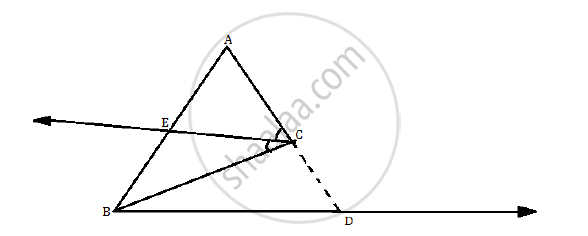# Prove that the Angle Bisector of a Triangle Divides the Side Opposite to the Angle in the Ratio of the Remaining Sides. - Geometry

Prove that the angle bisector of a triangle divides the side opposite to the angle in the ratio of the remaining sides.

#### Solution

Consider ΔABC,

Observe the following figure.CE bisects ∠ACB.

Draw a line parallel to ray CE passing through the point B.

Extend AC so as to intersect it at D.

Line CE is parallel to line BD and AD is the transversal.

∴ ∠ACE = ∠CDB [corresponding angles]      ….(1)

Now consider BC as the transversal.

∴ ∠ECB = ∠CBD [alternate angles]              ….(2)

But ∠ACE = ∠ECB [given]                           ….(3)

∴ ∠CBD = ∠CDB [from (1), (2) and (3)

In ΔCBD, side CB = side CD [sides opposite to equal angles]

∴ CB = CD                                                  ….(4)

Now in DABD, seg EC || side BD          [construction]

therefore"AE"/"EB"="AC"/"CD"                            [B.P.T]        .............(5)

therefore"AE"/"EB"="AC"/"CB"                             [from equations (4) and (5)]

Thus, the angle bisector of a triangle divides the side opposite to the angle in the ratio of the remaining sides.

Concept: Similarity of Triangles
Is there an error in this question or solution?